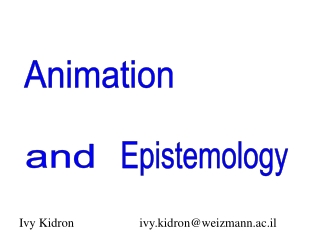DownloadDownload PresentationAnimation

# Animation

Download Presentation## Animation

- - - - - - - - - - - - - - - - - - - - - - - - - - - E N D - - - - - - - - - - - - - - - - - - - - - - - - - - -
##### Presentation Transcript

1. Animation Epistemology and Ivy Kidron ivy.kidron@weizmann.ac.il

2. The complexity of the instrumentation process with regard to animations

3. How animations might affect the teaching and learning of some subjects in mathematics?

4. Important role played by the usage of animations in students’ process and object understandings Using animations could reinforce some existing misconceptions or generate new misleading images.

5. Animation and generalization Exploring families of functions with parameters. Animation and visualization of dynamic processes Process of differentiation Convergence processes

6. Examples from a research on Approximation and Interpolation Calculus Laboratory from Visual Interpretation to Formal Reasoning Taught at the secondary/tertiary interface. Age 16 – 17, N = 84

7. The technological environment Symbolic tool web Numeric tool Graphics classnet Animation Communications possibilities The software (Wolfram Research) Mathematica

8. What is an animation? An animation is a sequence of pictures that you flip through quickly. If the pictures are related to each other in some sensible way, you get the illusion of motion. T. W. Gray and J. Glynn “Exploring Mathematics with Mathematica” (1991)

9. Click to view the animation Families of functions with parameters: Animated Graphics a = -3, -2, -1, 0, 1, 2, 3

10. Click to view the animation Periodic functions n = 1, 2, 3, 4, 5

11. Click to view the animation Visualization of dynamic processes The process of differentiation in red in green

12. Discrete - Continuous By means of animation , visualization of the process for decreasing values of h (a finite number)

13. The complexity of the instrumentation process Visualization of the process of differentiation The dynamic image produced by the animation could reinforce can be the misconception: very small. replaced by for (how much small?)

14. The complexity of the instrumentation process Mathematica might be used in order to overcome some of the misleading images: Graphically we can plot the difference - for Numericallywe can calculate values of the differencefor different x.

15. Visualization of dynamic processes Sin(x) and the approximating polynomials

16. Animation on the degree of the polynomial

17. Animation on the domain Sin(x) and We fix n = 5 and we change the domain i is decreasing from 4 to 1 with step -1

18. Animation on the domain

19. The Lagrange remainder ( For any in f(x)’s domain for some c . between 0 and

20. The Lagrange remainder Expansion of f(x) = Sin(x) at x = 0 up to exponent 5: The error is for some c between 0 and the current x value.

21. The (absolute value of the) error as a function of x and c an upper estimate

22. Click to view the animation Animation illustrating f(x) = Sin(x) n = 3, 5, 7, 9

23. Animation illustrating f(x) = Sin(x) We fix n = 5 and we change the domain i is decreasing from 4 to 1 with step -1

24. The complexity of the instrumentation process Former animations were present in the students’ minds when they were generating new animations, and sometimes it was a source of conflict. The students have seen by 2- dimensional animations that the different approximating polynomials “shared more ink” with the function when the degree of the Taylor polynomial increased. In sin(x)’ example, when n was increasing the error was steadily decreasing for every n. This was not the case for other examples such as Tami’s example.

25. Analysis of Tami's example f(x) = Sin(x) Cos(x) for as a function of x and c n = 1, 2, 3, 4, 5 All the graphs were surprising!

26. The way Tami used Mathematica in order to check this surprising situation Tami expanded f(x) in power series and revised the visual pictures of the polynomials approximating better f(x) (in red) as n increases. f(x)=sin(x) cos(x) and the different polynomials which approximate it

27. "dynamic" plot of f(x) and the approximating polynomials Tami"it seems that the polynomials better approach the function when n is bigger. It is strange! When we looked at the animation of the upper estimate of the remainder of Lagrange, the result was different: The maximal error was getting bigger when n increased." The surprising effect brought Tami to research the exact meaning of approximating better f(x) as n is increasing.

28. Discrete - Continuous The written test Visualization of the process described by the formal definition of limit Ability to translate visual pictures to analytical language N = 84, 81% proceeded step by step through a discrete sequence of finding the appropriate and were aware that the process is infinite. To every there is (sequential thinking)

29. Difficulties in reversing the order worked in the laboratory (beginning with domain and finding the error). is not dependent on . is dependent on is not dependent on the error, since is fixing the error: the nearer we approach the point x=0 about which the function was expanded, the smaller is the error. 68% expressed the formal definition: to every positive number, there is a positive number such that…

30. back

31. back

32. back

33. back